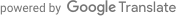## 数学笔记

$\hat{\bar{y}} = \frac{\sum_{i \in s} y_i}{n} \qquad(3.1)$

$\hat{\bar{y}} = \frac{1}{N} \sum_{i \in s} \frac{y_i}{\pi_i} \qquad(3.2)$

$\hat{\bar{y}} = \frac{1}{N} \sum_{i \in s} w_i y_i \qquad(3.3)$

$\pi_i = \frac{n_h}{N_h} \mbox{ for all } i \in h \qquad(3.4)$

$\hat{\bar{y}}_{post} = \sum_{h \in H} \frac{N_h}{N} \hat{\bar{y}}_h \qquad(3.5)$

$pr(i \in r) = \pi_i \phi_i \qquad(3.6)$

$\mbox{bias of sample mean} = \frac{cor(\phi, y) S(y) S(\phi)}{\bar{\phi}} \qquad(3.7)$

• 失业状况没有变化$$(S(y) = 0)$$
• 响应倾向没有变化$$(S(\phi) = 0)$$
• 反应倾向与失业状态之间没有相关性$$(cor(\phi, y) = 0)$$

$bias(\hat{\bar{y}}_{post}) = \frac{1}{N} \sum_{h=1}^H \frac{N_h cor(\phi, y)^{(h)} S(y)^{(h)} S(\phi)^{(h)}}{\bar{\phi}^{(h)}} \qquad(3.8)$

Bethlehem (2010)将关于后分层的许多上述推导扩展到包括无回应和覆盖误差。除了后分层，其他处理非概率样本的技术 - 以及覆盖误差和无响应的概率样本 - 包括样本匹配(Ansolabehere and Rivers 2013; ??? ) ，倾向得分加权(Lee 2006; Schonlau et al. 2009)和校准(Lee and Valliant 2009) 。这些技术中的一个共同主题是使用辅助信息。